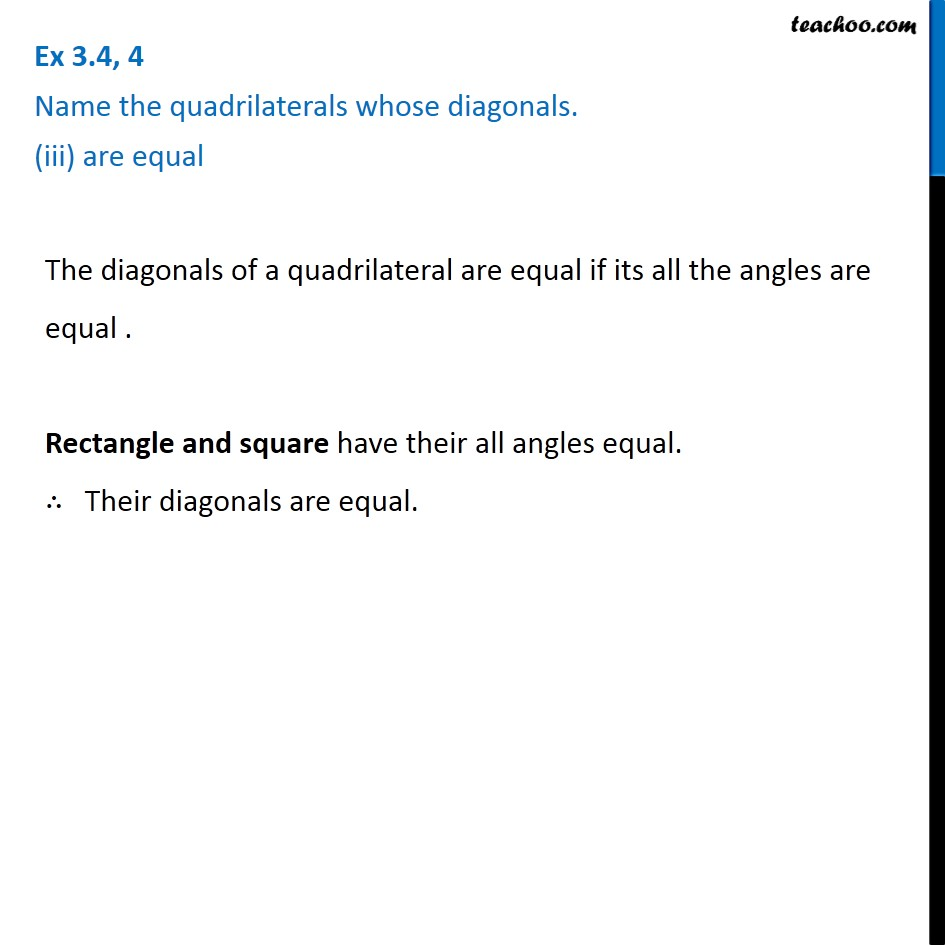Ex 3.4

Chapter 3 Class 8 Understanding Quadrilaterals
Serial order wiseLearn in your speed, with individual attention - Teachoo Maths 1-on-1 Class

### Transcript

Ex 3.4, 4 Name the quadrilaterals whose diagonals. (iii) are equal The diagonals of a quadrilateral are equal if its all the angles are equal . Rectangle and square have their all angles equal. ∴ Their diagonals are equal.